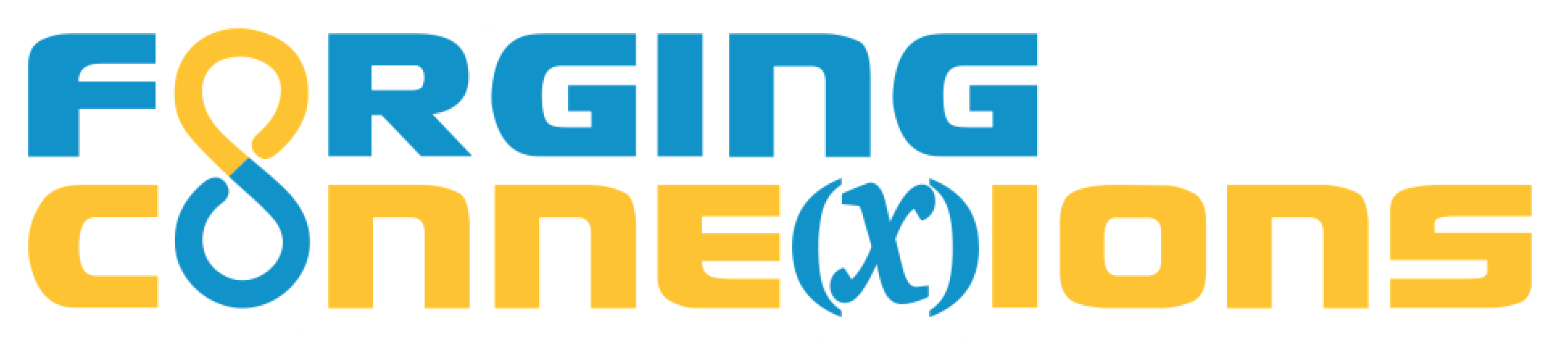Lesson Template

1 Warm-Up

The Warm-Up section is normally visible by default, so includes the fcVisible class in the fcSectionBody and uses data-url instead of data-delayed to specify the sketch json.

2 Mathematical Notation

Specify the jaxContainer class if your div uses math formatting, and specify the twoColumn class to format it in columns.

We use a system called AsciiMath or AsciiMathML for mathematical formatting. Each math expression is delimited by backticks (right under the escape key on your keyboard), and can display various mathematical symbols, formulas, etc.

Here are a few examples:

For this math Type this (between backticks)
overline(AB) overline(AB)
vec(AB) vec(AB)
stackrel harr (AB) stackrel harr (AB)
stackrel harr (AB) ∥ overline(CD) stackrel harr (AB) ∥ overline(CD)
stackrel harr (AB)\ ⟂\ overline(CD) stackrel harr (AB)\ ⟂\ overline(CD) (The "\ " adds a space.)
angleABC /_ABC or angleABC
triABC triABC
circleAB circleAB
(x+2)/(y-3) (x+2)/(y-3)
int_0^1 x^2\ dx int_0^1 x^2\ dx (The "\ " adds a space before dx.)
sum_(i=1)^n n = (n*(n + 1))/2 sum_(i=1)^n n = (n*(n + 1))/2
x = (-b +- sqrt(b^2 - 4ac))/(2a) x = (-b +- sqrt(b^2 - 4ac))/(2a)

The circle (circle) and triangle (tri) symbols are not standard AsciiMath. Math layout tools for the web have historically neglected elementary geometry in favor of a multitude of operator symbols, so neither TeX, LaTeX, nor MathML has standard symbols for geometric circles. With the help of Davide Cervone from MathJax, I have put in place a custom configuration for MathJax that makes these symbols work for us.

This section has it all: a two-column intro, two subsections each with its own sketch, and an embedded video in the section body itself.

Text (possibly in a div of class jaxContainer and/or twoColumn) can go above or below the sketch.

Hint videos coming soon: Page 1 Page 2

Hint videos coming soon: Page 1 Page 2

Below is an embedded video with a poster. You can use embedded videos, or videos that appear (like the hint videos) in a modal window.

4 Objectives

This page supports the Rotate Family lesson from the Introducing Geometric Transformations as Functions unit. You will construct rotation functions, manipulate and observe them, and learn how this function family behaves.

By the end of this lesson, you’ll be able to perform these actions and answer these questions:

• Item 1 This is the first of some number of items.

Early items in the above list should be as specifically targeted as possible to things students actually do during the lesson.

Later items can be more general, and more abstract in nature.

The Fine Print

Requirements:

These activities require web access using a browser that supports HTML5 and JavaScript. (That means almost any current browser.) No purchase is required, and there’s no advertising anywhere.

Release Information

Update History:

Record every major revision, in reverse chronological order

14 Feb 2018: Simplified this template.
24 Jan 2018: Created this lesson template.